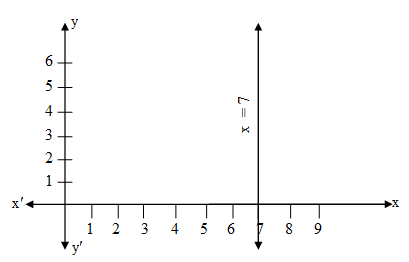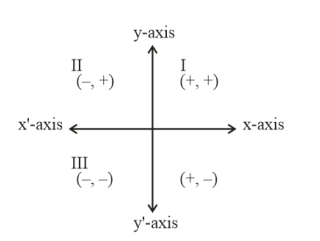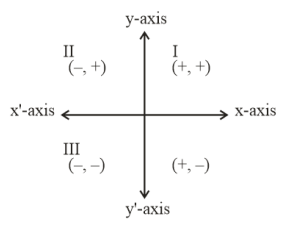Filter By

## All Questions

#### The line represented by x = 7 is parallel to the x–axis. Justify whether the statement is true or not.

Solution:From the above graph it is clear that x = 7 is not parallel to x axis but it is parallel to y-axis.Hence the given statement is false.

#### For all real values of c, the pair of equations x– 2y = 8, 5x – 10y = c have a unique solution. Justify whether it is true or false.

Solution:
Equation are x – 2y = 8
5x – 10y = c
Here a1 = 1, b1 = –2, c1 = –8
a2 = 5, b2 = –10, c2 = –c

For unique solution  but here

Hence the given statement is false.

## Crack CUET with india's "Best Teachers"

• HD Video Lectures
• Unlimited Mock Tests
• Faculty Support#### For the pair of equations x + 3y = –7 ,2x + 6y = 14to have infinitely many solutions, the value of  should be 1. Is the statement true?Give reasons.

Solution:
Here equations are x + 3y = –7
2x + 6y = 14
a1 = , b1 = 3, c1 = 7
a2 = 2, b2 = 6, c2 = –14
The equation have infinitely many solution

Here we can see that

Hence no value of  exist because it is given that equation has infinitely many solutions
Hence the statement is false.

#### Are the following pair of linear equations consistent? Justify your answer.(i) –3x– 4y = 12 ; 4y + 3x = 12(ii)  ; (iii) 2ax + by = a; 4ax + 2by = 2a ; a, b ≠ 0 (iv) x + 3y = 11 ; 4x + 12y = 22

Solution: (i)
Equation are : –3x – 4y = 12
4y + 3x = 12
In equation
–3x – 4y – 12 = 0
a1 = –3; b1 = –4 ; c1 = –12
In equation
4y + 3x – 12 = 0
a2 = 3 ; b2 = 4; c2 = –12

For consistency either  but here

Hence the pair of linear equations is not consistent.
Solution: (ii)
Equation are :

In equation

a1= ; b1 = –1 ; c1 =
In equation

a2 = 1/5 ; b2 = – 3; c2 = –1/6

For consistency either  also here

Hence given equations are consistent.
Solution: (iii)
Equation are:   2ax + by = a
4ax + 2by = 2a
In equation
2ax + by – a = 0
a1 = 2a; b1 = b; c1 = –a
In equation
4ax + 2by – 2a = 0
a2 = 4a ; b2 = 2b; c2 = –2a

For consistency either

Also, here

Hence given equations are consistent
Solution: (iv)

Given, equations are   x + 3 y = 11
4x + 12y =22
In equation
x + 3y = 11
a1 = 1; b1 = 3; c1 = –11
In equation
4x + 12y = 22
a2 = 4 ; b2 = 12; c2 = –22

For consistency either  but here

Hence given equations are not consistent.

## Crack NEET with "AI Coach"

• HD Video Lectures
• Unlimited Mock Tests
• Faculty Support#### Do the following equations represent a pair of coincident lines? Justify your answer.(i); 7x + 3y = 7 (ii) –2x – 3y = 1 ; 6y + 4x = –2 (iii)

(i)Solution:
Equation are 3x + y = 3
7x + 3y = 7
In equation
3x + y – 3 = 0
a1 = 3 ; b1 =  ; c1 = –3
In equation
7x + 3y – 7 = 0
a2 = 7 ; b2 = 3; c2 = –7

For coincident lines  but here

Hence the given pair of equations does not represent a pair of coincident lines.

(ii)Solution:

Equation are   –2x – 3y = 1
4x + 6y = –2
In equation
–2x – 3y  – 1 = 0
a1 = –2 ; b1 = –3 ; c1 = –1
In equation
4x + 6y + 2 = 0
a2 = 4 ; b2 = 6; c2 = 2

For coincident lines  also here
Hence the given pair of equation represents a pair of coincident lines.

Solution: (iii)
Equation are

4x + 8y + = 0
In equation

a1 = 1/2 ; b1 = 1 ; c1 =
In equation
4x + 8y +  =  0
a2 = 4 ; b2 = 8; c2 =

For coincident lines but here

Hence the given pair of equations does not represent a pair of coincident lines.

#### Do the following pair of linear equations have no solution? Justify your answer.(i) 2x + 4y = 3 ; 12y + 6x = 6 (ii) x = 2y; y = 2x (iii) 3x + y – 3 = 0 ;

(i)Solution:
Equations are 2x + 4y = 3
12y + 6x = 6
In equation 2x + 4y – 3 = 0
a1 = 2, b1 = 4, c1 = –3
In equation 12y + 6x – 6 = 0
a2 = 6, b2 = 12, c2 = –6

For no solution  also, Here we have

Hence the given equation have no solution.
(ii)Solution:
Equations are x – 2y = 0
–2x + y = 0
In equation
x – 2y = 0
a1 = 1, b1 = –2, c1 = 0
In equation
–2x + y = 0
a2 = –2, b2 = 1, c2 = 0

For no solution  but here

Hence the given pair of equations a unique solution.

(iii)Solution:
Equations are  3x + y = 3

In equation
3x + y – 3 = 0
a1 = 3, b1 = 1, c1 = –3
In equation

a2 = 2, b2= , c2 = –2

For no solution  but here

Hence the given pair of equations have infinity many solutions.

## Crack JEE Main with "AI Coach"

• HD Video Lectures
• Unlimited Mock Tests
• Faculty Support#### Write whether the following statements are True or False? Justify your answer. (-1, 7) is a point in the II quadrant.

Solution.We know that signs in II quadrant are (-, +) and here we have the point as (-1, 7)
i.e., x-coordinate is negative and y-coordinate is positive.
Therefore the given statement is True.

#### Write whether the following statements are True or False? Justify your answer. A point lies on y-axis at a distance of 2 units from the x-axis. Its coordinates are (2, 0).

Solution.

We know that points that lie on the y-axis have coordinate in the form (0, y).
So we can say that x-coordinate should be zero.
The distance from x-axis will be equal to its y-coordinate.
So the point will be (0, 2)
But here the point is given as (2, 0) so the statement is false.

## Crack CUET with india's "Best Teachers"

• HD Video Lectures
• Unlimited Mock Tests
• Faculty Support#### Write whether the following statements are True or False? Justify your answer. The coordinates of a point whose ordinate is -1/2 and abscissa is 1 are -1/2 , 1.

Solution.

The abscissa is the x-axis (horizontal) coordinate
The ordinate is the y-axis (vertical) coordinate
Here ordinate is -1/2 and abscissa is 1.
So the coordinates are (1, -1/2) and not (-1/2, 1) .
Therefore the given statement is False.

#### Write whether the following statements are True or False? Justify your answer. Points (1, -1) and (-1. 1) lie in the same quadrant.

Solution.False, point (1, -1) and (-1. 1) lie in the different quadrants.
Point (1, -1) lies in the IV quadrant and point (-1. 1) lies in the II quadrant.
Therefore the given statement is False.